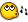# Help With If In Loop## Recommended PostsHi, I'm trying to create IF in a loop

Like:

<start>

A or B? <A>

execute A

execute B

execute A

execute B

... inifinte

I wrote a script that should do it, but I will get the error that the infinite loop wont work, cant find Wend / Until ... Why won't it work? Without the If or without the do loop it will workDim \$l

Dim \$d

Dim \$i

MsgBox(4096, "Start", "Start"&@CRLF, 10)

\$l = InputBox("L?", "L?", "1", "", -1, -1, 0, 0)

Sleep(10000)

Do

If \$l=1 Then

For \$i=1 to 10

MsgBox(4096, "case", "1"&@CRLF, 10)

Next

\$l=2

Elseif \$l=2 Then

For \$i=1 to 10

MsgBox(4096, "case", "2"&@CRLF, 10)

Next

\$l=1

Else

Exit

EndIf

Until \$d=-1

Edited by DJBeri
##### Share on other sites

a few problems but try this:

```Dim \$l
MsgBox(4096, "Start", "Start"&@CRLF, 10)
;Sleep(10000)
Do
\$l = InputBox("L?", "L?", "1", "", -1, -1, 0, 0)
If \$l=1 Then
MsgBox(4096, "case", "1"&@CRLF, 10)
\$l=2
Elseif \$l=2 Then
MsgBox(4096, "case", "2"&@CRLF, 10)
\$l=1
Else
Exit
EndIf
Until \$l=-1```

Until \$d=-1 what is d? is \$d called out somewhere else?

Did you want it to change? if so then here it is but you can't actually exit once it starts:

```Dim \$l
MsgBox(4096, "Start", "Start"&@CRLF, 10)
\$l = InputBox("L?", "L?", "1", "", -1, -1, 0, 0)
Do
If \$l=1 Then
MsgBox(4096, "case", "1"&@CRLF, 10)
\$l=2
Elseif \$l=2 Then
MsgBox(4096, "case", "2"&@CRLF, 10)
\$l=1
Else
Exit
EndIf
Until \$l=-1```
Edited by scriptkitty

AutoIt3, the MACGYVER Pocket Knife for computers.

## Create an account

Register a new account

• ### Recently Browsing   0 members

×

• Wiki

• Back

• #### Beta

• Git
• FAQ
×
• Create New...